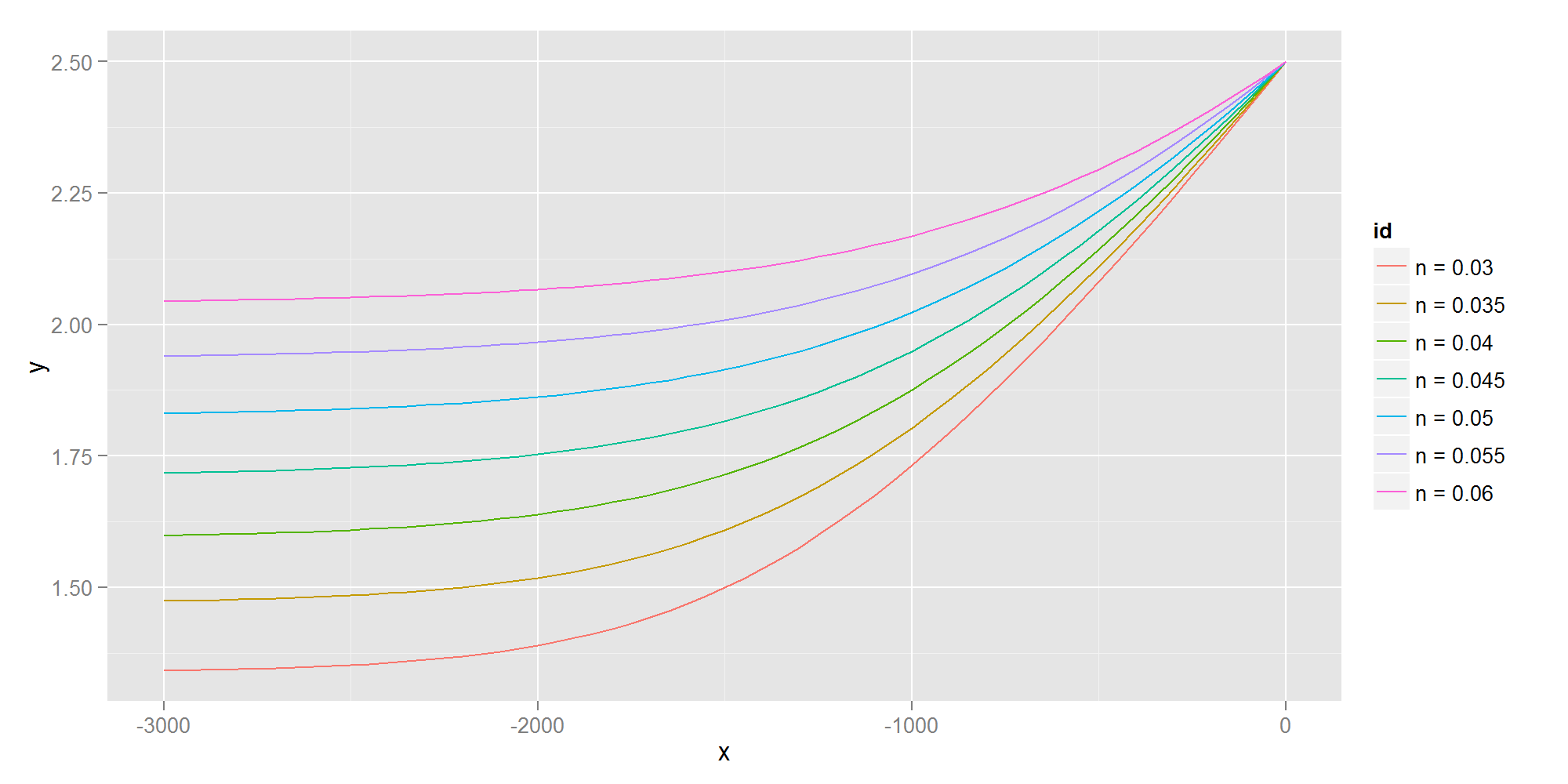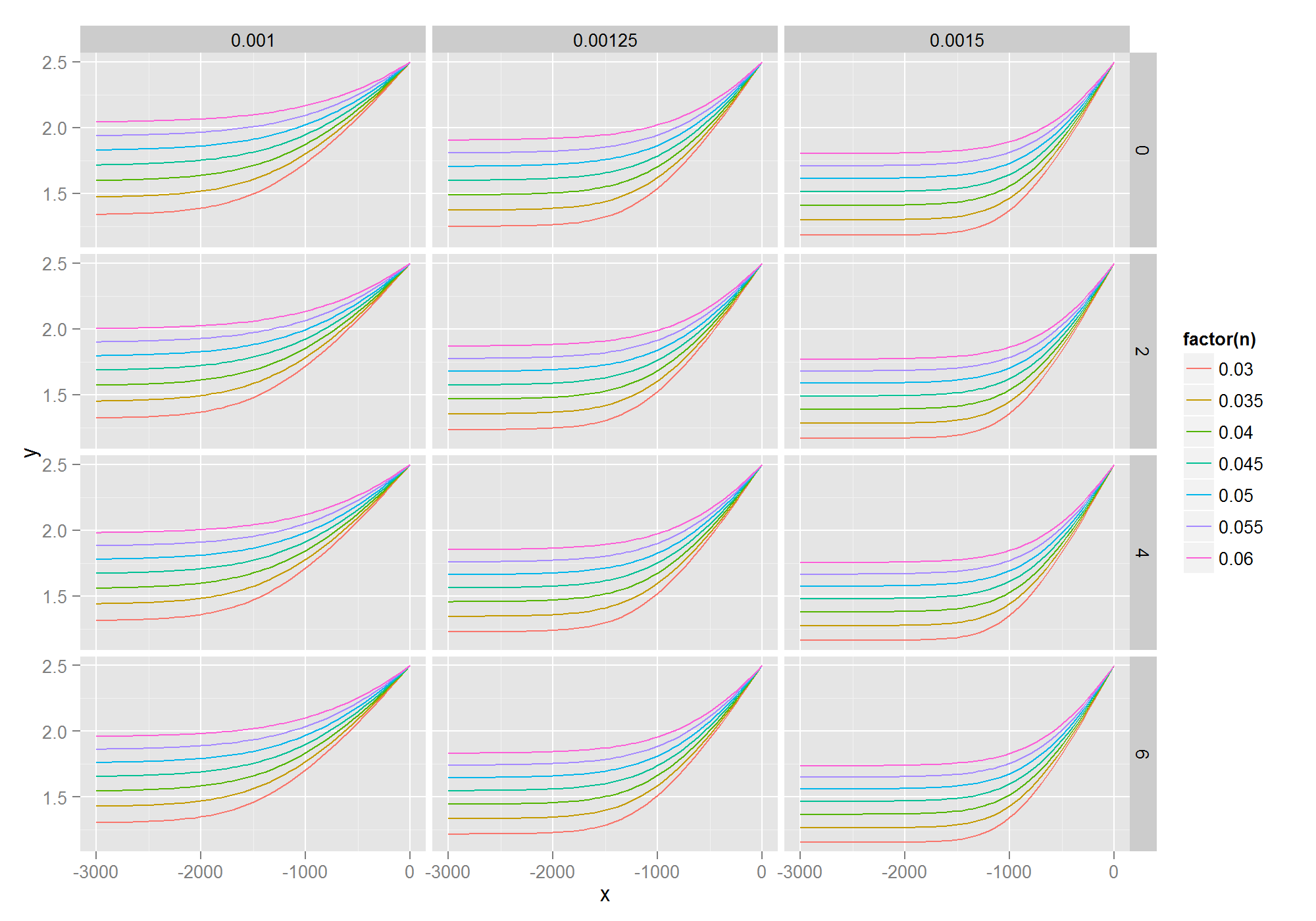# Quickstart for reval

## Introduction

This vignette will get you stated using `reval`. Additional examples are available in the `evalmany` function documentation.

## Example: Channel design sensitivity analysis

In-stream structures such as dams, weirs and culverts modify flows in a river. In large rivers with tranquil flows, such structures can affect the river stage (water depth) many miles upstream. These water surface profiles or “backwater curves” can be modelled using well-understood hydraulic relationships. One important parameter—a coefficient representing the texture or “roughness” of the river bed—is empirical and cannot be easily measured. Therefore it is often important for engineers to compute these backwater curves for a range of roughness values in order to establish confidence limits for planning and management purposes.

The `rivr` package provides the function `compute_profile` for modelling backwater curves in prismatic channels given a known water depth at a specified location. Computing a single profile would look something like this:

``````# install.packages('rivr')
require(rivr)
myprofile = compute_profile(So = 0.001, n = 0.045, Q = 250, y0 = 2.5, Cm = 1.486,
g = 32.2, B = 100, SS = 0, stepdist = 50, totaldist = 3000)
``````
``````##      x    z        y        v        A           Sf        E        Fr
## 1    0 0.00 2.500000 1.000000 250.0000 0.0002884384 2.515528 0.1114556
## 2  -50 0.05 2.464316 1.014480 246.4316 0.0003023231 2.530297 0.1138852
## 3 -100 0.10 2.429331 1.029090 242.9331 0.0003167995 2.545775 0.1163542
## 4 -150 0.15 2.395073 1.043809 239.5073 0.0003318679 2.561992 0.1188595
## 5 -200 0.20 2.361575 1.058616 236.1575 0.0003475244 2.578977 0.1213974
## 6 -250 0.25 2.328865 1.073484 232.8865 0.0003637600 2.596759 0.1239640
``````

In order to perform a sensitivity analysis on the effect of the roughness parameter on the backwater curve, we need to loop through a series of roughness values (the argument “n”) and compile the results for comparison. This could be accomplished with a simple for loop:

``````# loop through values of n
ns = seq(0.03, 0.06, by = 0.005)
results = vector("list", length = length(ns))
for (i in 1:length(ns)) {
results[[i]] = compute_profile(So = 0.001, n = ns[i], Q = 250, y0 = 2.5,
Cm = 1.486, g = 32.2, B = 100, SS = 0, stepdist = 50, totaldist = 3000)
# add an identifier to the result
results[[i]]["n"] = ns[i]
}
# combine outputs
combined = do.call(rbind.data.frame, results)
``````

That wasn't too bad, but it's even easier with `reval`. We can replace that entire chunk of code with a single call to `evalmany`:

``````results = evalmany(compute_profile, n = seq(0.03, 0.06, by = 0.005), default.args = list(So = 0.001,
Q = 250, y0 = 2.5, Cm = 1.486, g = 32.2, B = 100, SS = 0, stepdist = 50,
totaldist = 3000))
``````Other factors can also influence the water surface profile, such as the channel slope and the angle of the channel walls. If we wanted to perform a sensitivity analysis based on these parameters as well, we would need to use a set of nested loops or add code to organize the sequence of parameter sets to evaluate. This would impact readability and make code maintenance a hassle. In contrast, performing a multi-parameter sensitivity analysis with `reval` is trivial. Here we use `evalmany` to evaluate all possible permutations of the three parameters. When performing more intensive computations, parallel processing capabilities from the `doParallel` package can be leveraged using the argument `clusters`.

``````results = evalmany(compute_profile, n = seq(0.03, 0.06, by = 0.005), So = seq(0.001,
0.0015, by = 0.00025), SS = seq(0, 6, by = 2), default.args = list(Q = 250,
y0 = 2.5, Cm = 1.486, g = 32.2, B = 100, stepdist = 50, totaldist = 3000),
method = "permute", collate.id = "multi", clusters = 2, packages = "rivr")
``````

The `collate.id` argument is particularly useful for plotting with `ggplot2` and for subsetting data with `dplyr`.

``````require(ggplot2)
ggplot(results, aes(x = x, y = y, color = factor(n))) + geom_line() + facet_grid(SS ~
So)
````````````require(dplyr)
filter(results, n == 0.045, So == 0.0015, SS == 2)
``````
x z y v A Sf E Fr n So SS
0 0.000 2.500000 0.9523810 262.5000 0.0002646 2.514084 0.1086462 0.045 0.0015 2
-50 0.075 2.438050 0.9777343 255.6932 0.0002878 2.527894 0.1128860 0.045 0.0015 2
-100 0.150 2.377273 1.0038946 249.0301 0.0003133 2.542922 0.1173166 0.045 0.0015 2
-150 0.225 2.317781 1.0308330 242.5223 0.0003411 2.559281 0.1219375 0.045 0.0015 2
-200 0.300 2.259696 1.0585056 236.1820 0.0003714 2.577094 0.1267454 0.045 0.0015 2
-250 0.375 2.203146 1.0868510 230.0223 0.0004044 2.596488 0.1317336 0.045 0.0015 2

In this case `compute_profile` outputs a dataframe and collating works as expected. When the input function does not produce a dataframe, `evalmany` will attempt to coerce the output using `as.data.frame`. An optional function to further manipulate the raw output prior to coercion to a data frame can be passed via the argument `collate.fun`. Alternatively, the raw results can be output as a named list with `collate = FALSE`.

## Still confused?

Check out the `evalmany` function documentation for more examples.